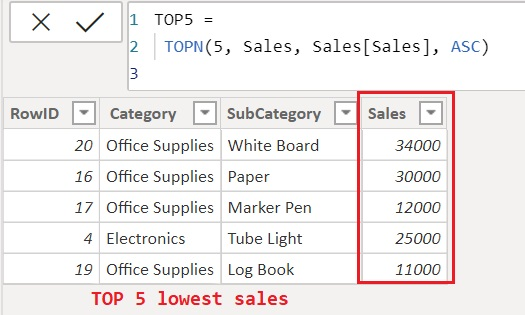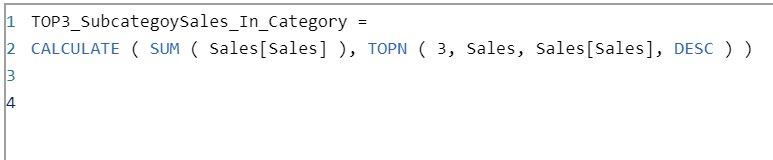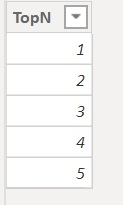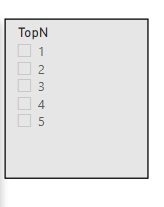Home » TOPN Function DAX

# TOPN Function DAXTOPN function is a Power BI table manipulation function in DAX which returns the top n records from table.

• If you specify the number 0 to TOPN function or any less number then TOPN returns an empty table.
• TOPN function does not guarantee any sort order for the results.
• If there is a tie for order_by values at the N-th row of the table then all tied rows are returned. Then, when there are ties at the N-th row the function might return more than n rows.

SYNTAX

`TOPN(<n_value>, <table>, <orderBy_expression>, [<order>[, <orderBy_expression>, [<order>]]…])`

n_value It specifies the number of rows to return. A single scalar value will be returned where the expression will evaluate multiple rows.

table Is the table from where you would like to extract the TOPN rows.

orderBy_expression Any DAX expression where the result value of the expression will sort and evaluate each row of the table.

order Is used to your expression either ascending (ASC, or TRUE, or 1) or descending (DESC, or FALSE, or 0) order. It is an optional.

Lets look at an example of TOPN function.

#### Basic use of TOPN Function

Lets take a look at below table named Sales, which contains Sales by Category and Subcategory.Suppose, you want to see top 5 highest sales among all the sales records, then you can use TOPN function which allows you to see the top n rows from table.

Following DAX measure uses a TOPN function which returns a new table containing a rows contains top 5 highest sales among all the sales from Sales table.

```TOP5 =
TOPN(5,(Sales), Sales[Sales], DESC)```As you can see, it returns top 5 records with highest sales.

You will also note that although TOPN function returns the top 5 sales but does sort the order in descending order as it does not guarantee any sort order for the results.

Similarly to see the top 5 lowest sales records just provide the parameter order as ASC in above measure.

```TOP5 =
TOPN(5, Sales, Sales[Sales], ASC)```#### Some other ways of using TOPN function

Following example demonstrate, how you can use TOPN function to see the Total highest sales of TOP 3 sub category for each category in table.

Lets create a measure named TOP3_SubcategoySales_In_Category as following.

`TOP3_SubcategoySales_In_Category = CALCULATE ( SUM ( Sales[Sales] ), TOPN ( 3, Sales, Sales[Sales], DESC ) )`Lets commit the measure and drag into visual to see the output.

Now you can see the Total highest Sales of top 3 subcategory for each category.It is very useful in case, when you want to see the total contribution of top products against over all sales of products.

#### Implementing dynamic TOPN function

Following example demonstrate, how you can provide value to TOPN function dynamically for number of rows that you want to return.

As you have seen, in measure which returns Total sales of top three highest subcategory in each category only as a hardcoded value 3 is specified for parameter n_value in TOPN function.

The idea that based on the selected value in slicer, a TOPN function returns those number of rows from table.

Here we have a table named TOPNList, which contains a number starting from 1 to 5.Lets take a slicer and loads with values from table TOPNList.Now create a measure that will take a value selected in slicer and used in TOPN function as shown below.

`TOP_SubcategoySales_In_Category =`
```VAR get_n_value = SELECTEDVALUE ( TOPList[TopN] )
RETURN   CALCULATE (
SUM ( Sales[Sales] ),
TOPN ( get_n_value, Sales, Sales[Sales], DESC )
)```once you commit the measure, Lets see the total sales of TOP 2 highest subcategory for each category.

You can see it, returns the result accordingly, similarly for TOP 3 highest subcategory for each category.Table Manipulation functions

SUMMARIZE

SUMMARIZECOLUMNS

VALUES

DATATABLE